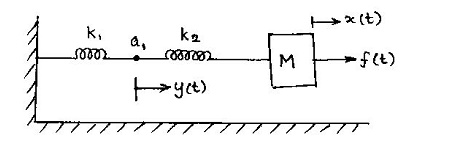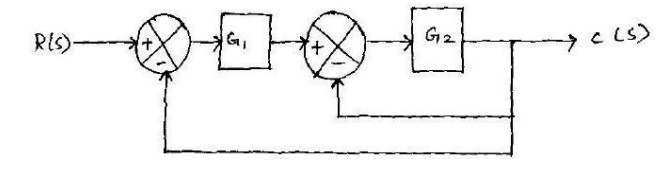Home | | Control System Engineering | Tutorial Problems: Control Systems - Systems and their Representation

# Tutorial Problems: Control Systems - Systems and their Representation

Control Systems - Systems and their Representation - Tutorial Problems: Control Systems - Systems and their Representation

TUTORIAL PROBLEMS

CONTROL SYSTEM MODELING

1. In the system shown in figure below, R, L and C are electrical parameters while K, M and B are mechanical parameters. Find the transfer function X(S)/E1(S) for the system, where E1(t) is input voltage while x(t) is the output displacement.2. (i) A block diagram is shown below. Construct the equivalent signal flow graph and obtain C/R using Mason‘s formula.3. (ii) For the block diagram shown below, find the output C due to R and disturbance D.4. Write the differential equations governing the mechanical rotational system shown in figure. Draw the torque-voltage and torque-current electrical analogous circuits and verify by writing mesh and node equations.5. (i) Using block diagram reduction technique, find the closed loop transfer function C/R of the system whose block diagram is shown below.(ii) Construct the signal flow graph for the following set of simultaneous equations and obtain the overall transfer function using Mason‘s gain formula.

X2 = A21X1 + A23X3

X3 = A31X1 + A32X2 + A33X3

X4 = A42X2 + A43X3

6. (i) Consider the mechanical system shown below. Identify the variables and write the differential equations.(ii) Draw the torque-voltage electrical analogous circuit for the following mechanical system shown.(iii) Obtain the transfer function of the following electrical network.7. (i) For the signal flow graph shown below, find C(S)/R(S) by using Mason‘s gain formula.(ii) Find the transfer function C(S)/R(S) of block diagram shown below.8. (i) Reduce the block diagram to its canonical form and obtain C(S)/R(S).(ii)Give the comparison between block diagram and signal flow graph methods.

Determine the transfer function for the system having the block diagram as shown below(iii) Determine the transfer function of the network in the figure.9. Determine the transfer function of the transistor‘s hybrid model shown in figure using signal flow graph.Study Material, Lecturing Notes, Assignment, Reference, Wiki description explanation, brief detail
Control Systems : Systems and their Representation : Tutorial Problems: Control Systems - Systems and their Representation |